fTools Plugin¶

The goal of the fTools python plugin is to provide a one-stop resource for many common vector-based GIS tasks, without the need for additional software, libraries, or complex workarounds. It provides a growing suite of spatial data management and analysis functions that are both fast and functional.

fTools is now automatically installed and enabled in new versions of QGIS, and as with all plugins, it can be disabled and enabled using the Plugin Manager (See Section Loading a QGIS Core Plugin). When enabled, the fTools plugin adds a Vector menu to QGIS, providing functions ranging from Analysis and Research Tools to Geometry and Geoprocessing Tools, as well as several useful Data Management Tools.

Analysis tools¶

Icon Tool PurposeDistance Matrix Measure distances between two point layers, and output results as a) Square distance matrix, b) Linear distance matrix, or c) Summary of distances. Can limit distances to the k nearest features.Sum line length Calculate the total sum of line lengths for each polygon of a polygon vector layer.Points in polygon Count the number of points that occur in each polygon of an input polygon vector layer.List unique values List all unique values in an input vector layer fieldBasic statistic Compute basic statistics (mean, std dev, N, sum, CV) on an input field.Nearest Neighbor analysis Compute nearest neighbor statistics assess the level of clustering in a point vector layerMean coordinate(s) Compute either the normal or weighted mean center of an entire vector layer, or multiple features based on a unique ID field.Line intersections Locate intersections between lines, and output results as a point shapefile. Useful for locating road or stream intersections, ignores line intersections with length > 0.

Table Ftools 1: fTools Analysis tools

Research tools¶

Icon Tool Purpose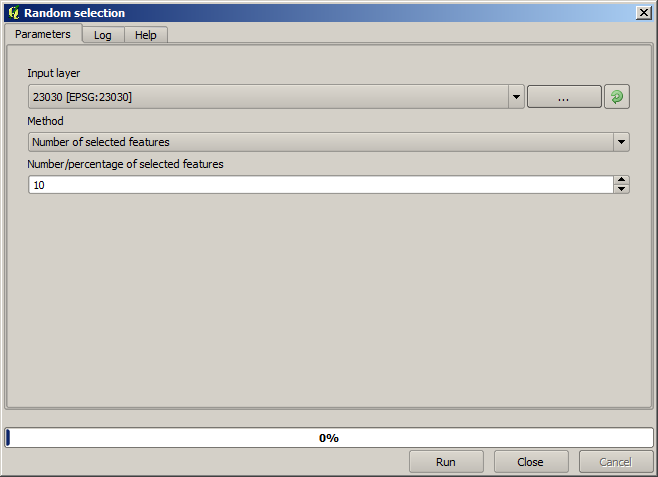Random selection Randomly select n number of features, or n percentage of featuresRandom selection within subsets Randomly select features within subsets based on a unique ID field.Random points Generate pseudo-random points over a given input layer.Regular points Generate a regular grid of points over a specified region and export them as a point shapefile.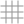Vector grid Generate a line or polygon grid based on user specified grid spacing.Select by location Select features based on their location relative to another layer to form a new selection, or add or subtract from the current selection.Polygon from layer extent Create a single rectangular polygon layer from the extent of an input raster or vector layer.

Table Ftools 2: fTools Research tools

Geoprocessing tools¶

Icon Tool Purpose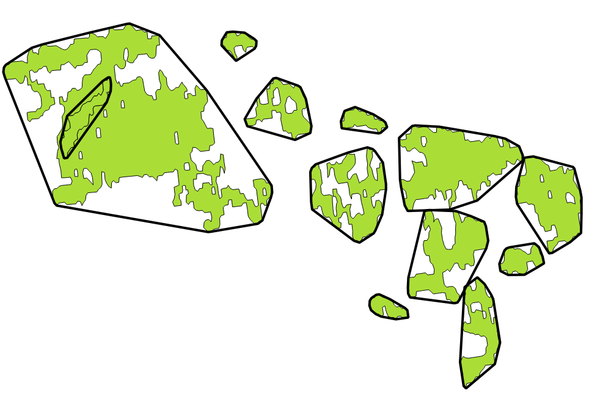Convex hull(s) Create minimum convex hull(s) for an input layer, or based on an ID field.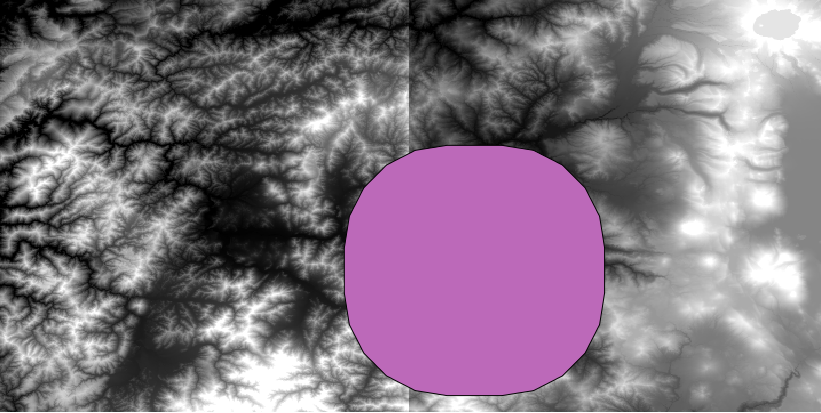Buffer(s) Create buffer(s) around features based on distance, or distance field.Intersect Overlay layers such that output contains areas where both layers intersect.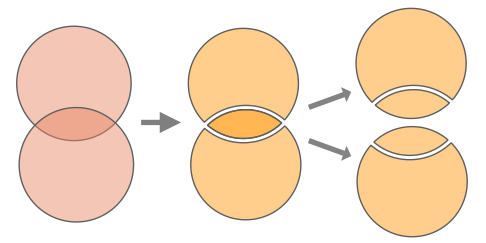Union Overlay layers such that output contains intersecting and non-intersecting areas.Symmetrical difference Overlay layers such that output contains those areas of the input and difference layers that do not intersect.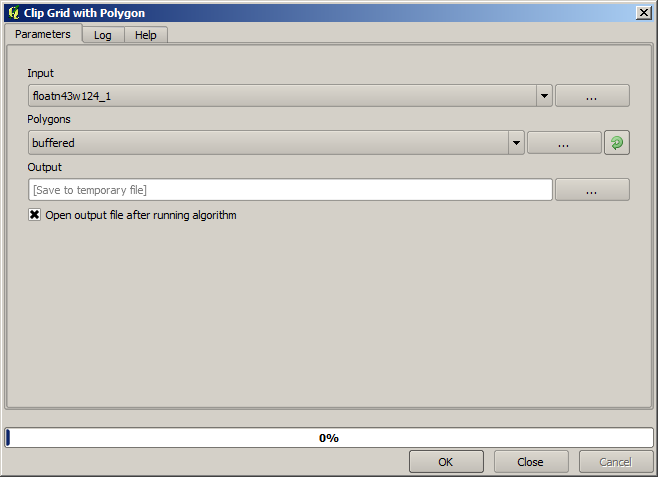Clip Overlay layers such that output contains areas that intersect the clip layer.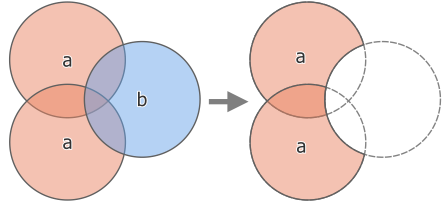Difference Overlay layers such that output contains areas not intersecting the clip layer.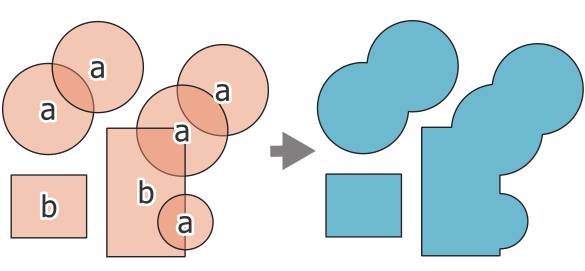Dissolve Merge features based on input field. All features with identical input values are combined to form one single feature.Eliminate sliver polygons Merges selected features with the neighbouring polygon with the largest area or largest common boundary.

Table Ftools 3: fTools Geoprocessing tools

Geometry tools¶

Icon Tool PurposeCheck geometry validity Check polygons for intersections, closed-holes, and fix node ordering.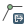Export/Add geometry columns Add vector layer geometry info to point (XCOORD, YCOORD), line (LENGTH), or polygon (AREA, PERIMETER) layer.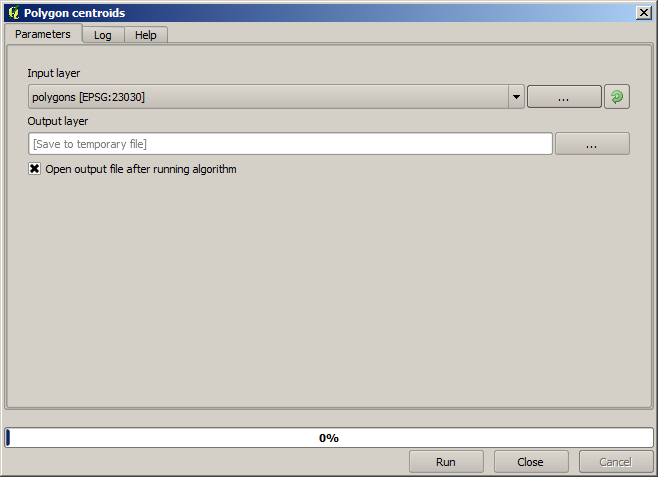Polygon centroids Calculate the true centroids for each polygon in an input polygon layer.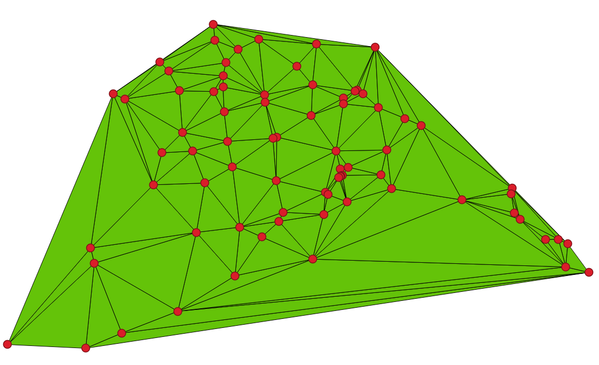Delaunay triangulation Calculate and output (as polygons) the delaunay triangulation of an input point vector layer.
Voronoi Polygons Calculate voronoi polygons of an input point vector layer.Simplify geometry Generalize lines or polygons with a modified Douglas-Peucker algorithm.
Densify geometry Densify lines or polygons by adding verticesMultipart to singleparts Convert multipart features to multiple singlepart features. Creates simple polygons and lines.Singleparts to multipart Merge multiple features to a single multipart feature based on a unique ID field.Polygons to lines Convert polygons to lines, multipart polygons to multiple singlepart lines.Lines to polygons Convert lines to polygons, multipart lines to multiple singlepart polygons.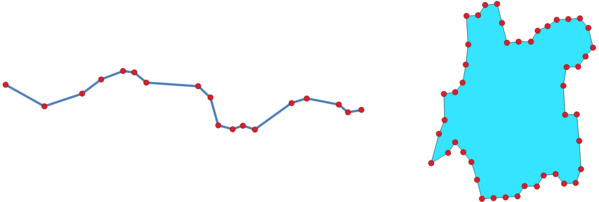Extract nodes Extract nodes from line and polygon layers and output them as points.

Table Ftools 4: fTools Geometry tools

Note

The Simplify geometry tool can be used to remove duplicate nodes in line and polygon geometries, just set the Simplify tolerance parameter to 0 and this will do the trick.

Data management tools¶

Icon Tool PurposeDefine current projection Specify the CRS for shapefiles whose CRS has not been defined.Join attributes by location Join additional attributes to vector layer based on spatial relationship. Attributes from one vector layer are appended to the attribute table of another layer and exported as a shapefile.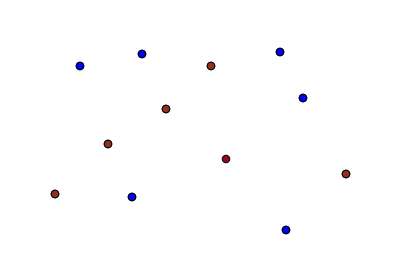Split vector layer Split input layer into multiple separate layers based on input field.Merge shapefiles to one Merge several shapefiles within a folder into a new shapefile based on the layer type (point, line, area).
Create spatial index Create a spatial index for OGR supported formats.

Table Ftools 5: fTools Data management tools